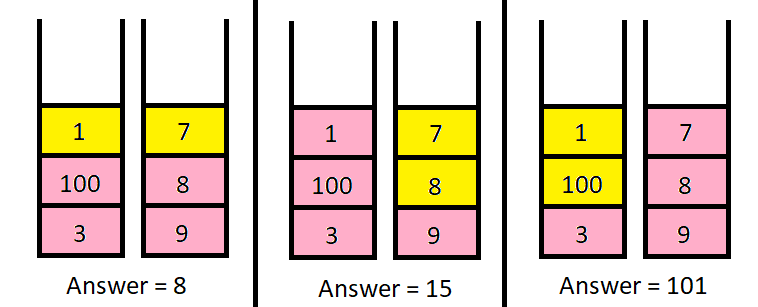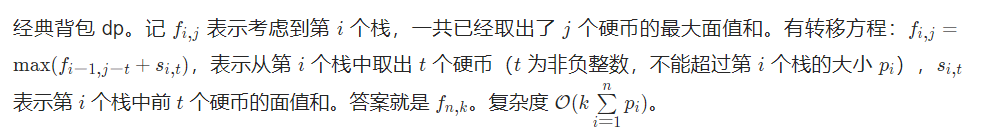## LeetCode第286场周赛题解

### 一、找出两数组的不同

answer 是 nums1 中所有 不 存在于 nums2 中的 不同 整数组成的列表。
answer 是 nums2 中所有 不 存在于 nums1 中的 不同 整数组成的列表。

输入：nums1 = [1,2,3], nums2 = [2,4,6]

输入：nums1 = [1,2,3,3], nums2 = [1,1,2,2]

nums2 中的每个整数都在 nums1 中出现，因此，answer = [] 。 

• 1 <= nums1.length, nums2.length <= 1000
• -1000 <= nums1[i], nums2[i] <= 1000

#### 题解：集合

C++代码：

class Solution {
public:
vector<vector<int>> findDifference(vector<int>& nums1, vector<int>& nums2) {
set<int> st1,st2;
int n=nums1.size();int m=nums2.size();
for(int i=0;i<n;i++){
st1.insert(nums1[i]);
}
for(int i=0;i<m;i++){
st2.insert(nums2[i]);
}
vector<vector<int> > ans(2, vector<int>());
for(auto x:st1){
if(st2.count(x)) continue;
ans.push_back(x);
}
for(auto x:st2){
if(st1.count(x)) continue;
ans.push_back(x);
}
return ans;
}
};

### 二、美化数组的最少删除数

nums.length 为偶数

输入：nums = [1,1,2,3,5]

输入：nums = [1,1,2,2,3,3]

• $1 <= nums.length <= 10^5$
• $0 <= nums[i] <= 10^5$

#### 题解：贪心

• 删除nums[i],num[i+1]前移，此时(i+1)%2==0需要和nums[i+2]比较
• 删除nums[i+1],i%2==0，同样需要和nums[i+2]比较

C++代码：

class Solution {
public:
int minDeletion(vector<int>& nums) {
int n=nums.size();
int cnt=0;
for(int i=0;i<n;i++){
int p=i-cnt;
if(i+1==n) break;
if(nums[i]==nums[i+1]&&p%2==0){
++cnt;
}
}
if((n-cnt)%2) ++cnt;
return cnt;
}
};

### 三、找到指定长度的回文数

输入：queries = [1,2,3,4,5,90], intLength = 3

101, 111, 121, 131, 141, 151, 161, 171, 181, 191, 201, ...

输入：queries = [2,4,6], intLength = 4

1001, 1111, 1221, 1331, 1441 和 1551 。

• $1 <= queries.length <= 5 * 10^4$
• $1 <= queries[i] <= 10^9$
• $1 <= intLength <= 15$

#### 题解：数学

intLength除2（intLength为奇数，则需要加1），由于是求回文数，于是我们只处理前一半数字即可。

• intLength=3，则len=intLength/2+1=2，用某一变量x存储后一半需要添加的数字，
• 于是我们只需要考虑前两位数字，最小为10，此时x为1
• 当我们需要计算此时第k小的回文数时，我们只需要对前面元素+(k-1)即可，例如求第3小的元素，此时前一半为10+2=12，后一半x为1，组合为121

• intLength=4，则len=intLength/2=2，用某一变量x存储后一半需要添加的数组
• 于是我们只考虑前两位数字，最小为10，此时x为1，
• 当我们需要计算此时第k小的回文数时，我们只需要对前面元素+(k-1)即可，例如求第3小的元素，此时前一半为10+2=12，后一半x为21，组合为1221

C++代码：

class Solution {
public:
typedef long long ll;
//得到后一半数字
ll get_num(int first_past,int flag){
//如果是奇数为，则需要只需考虑前一半的n-1位（n为前一半数字的位数）
if(flag) first_past/=10;
ll res=0;
while(first_past){
res=res*10+first_past%10;
first_past/=10;
}
return res;
}
vector<long long> kthPalindrome(vector<int>& queries, int intLength) {
vector<ll> ans;
int n=queries.size();
int m=intLength/2;
int flag=intLength%2?1:0;
if(flag) ++m;
for(int i=0;i<n;i++){
ll cnt=queries[i];
//前一半数字
ll first_past=pow(10,m-1)+cnt-1;
// cout<<first_past<<"---"<<pow(10,m)<<endl;
//如果前一半的数字都超过m位，则返回-1
if(first_past>=pow(10,m)){
ans.push_back(-1);continue;
}
//后一半数字
ll last_past=get_num(first_past,flag);
//当前第k小的数字
ll num=first_past*pow(10,m);
if(flag) num/=10;
num+=last_past;
ans.push_back(num);
}
return ans;
}
};

### 四、从栈中取出 K 个硬币的最大面值和输入：piles = [[1,100,3],[7,8,9]], k = 2

输入：piles = [,,,,,,[1,1,1,1,1,1,700]], k = 7

• $n == piles.length$
• $1 <= n <= 1000$
• $1 <= piles[i][j] <= 10^5$
• $1 <= k <= sum(piles[i].length) <= 2000$

#### 题解：分组背包dpC++代码：

class Solution {
public:
int inf=0x3f3f3f3f;
int maxValueOfCoins(vector<vector<int>>& piles, int k) {
int n=piles.size();
int dp;
memset(dp,-inf,sizeof(dp));
dp=0;
for(int i=1;i<=n;i++){
vector<int> sum;sum.push_back(0);
//前缀和
for(int x:piles[i-1]) sum.push_back(sum.back()+x);
for(int j=0;j<=k;j++){
for(int p=0;p<sum.size();p++){
if(j>=p) dp[i][j]=max(dp[i][j],dp[i-1][j-p]+sum[p]);
}
}
}
return dp[n][k];
}
};